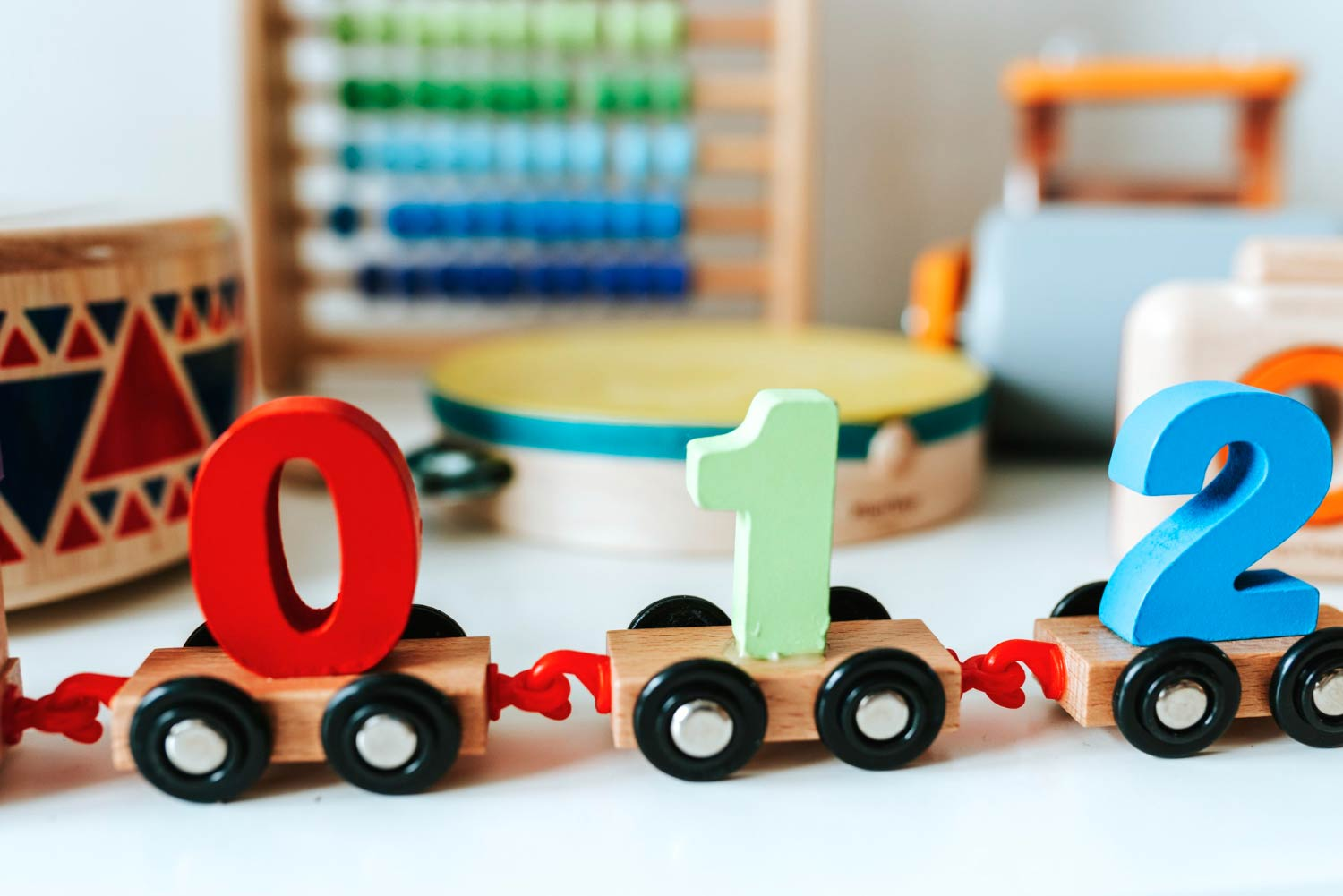If you are trying to learn about factors and multiples in your Primary 4 math class, knowing this rainbow method will come in handy. When it comes to finding the factors of a number, there are many ways of doing it, such as the listing method or guessing the factors. However, with the help of a consistent study habit, the easiest way is to use the rainbow method and this article will walk you through it and give you some tips on how to apply it during your child’s studies.### Rainbow Method Math, how to use it to find the factors of a number

Let’s say we are asked to find all the factors of 12. The first step that we are going to do is to write the numbers 1 and 12 with some space in between. We start with these 2 numbers because the easiest factor pair of any number is always 1 and that number itself. Once we are done, we’ll draw a bow that connects 1 and 12. This is the first part of rainbow method math. Next, we are going to move on to the next number, which is 2. Can we form a factor pair with it? Well, 2 x 6 = 12, so 2 and 6 is another factor pair of 12. We’ll write these numbers in the space with the smaller number on the left and the greater number on the right. Then, we’ll join them with another bow. After that, it’s time to move on to number 3. Can we multiply 3 by any number to get 12? Yes, we can! 3 x 4 = 12. That makes 3 and 4 a factor pair of 12. Let’s put that in between 2 and 6. That’s our third bow. When we look at the next number, we notice that 4 x 3 is going to give us the same factor pair as what we had earlier. This indicates that we’ve found all the factors of 12 and we can stop here. The rainbow factor pairs of 12 are 1 and 12, 2 and 6 and 3 and 4. Looking at the factor rainbow, we can tell that the factors of 12 are 1, 2, 3, 4, 6 and 12. Let’s try the math rainbow method with another example.

#### Try to find the factors of 30 using the Rainbow Method Math!

Can you remember the steps to do that using the math rainbow method?

Any number is equal to 1 multiplied by itself. So the first factor pair of 30 is 1 and 30.

### Step 2: Keep finding factor pairs by going down the numbers

We go down to the next number, which is 2. 2 x what number = 30? 2 x 15 equals to 30, which makes 2 and 15 the next factor pair.

### Step 3: Repeat until you’ve run out of numbers

The next pair of factors that we find is 3 and 10 because 3 x 10 = 30. Since we can’t get a factor pair with 4, we’ll continue with 5 x 6, which gives us 5 and 6 as another factor pair. And we’re done! What are the rainbow factor pairs of 30? They are 1 and 20, 2 and 15, 3 and 10 and 5 and 6. The factors of 30 are 1, 2, 3, 5, 6, 10, 15 and 30.

Now that we’ve seen how the rainbow method math works and how we can use it for finding and organising all the factors of a given number, try using this method for your math homework!

### One Comment

•annisa says:

Nicee!